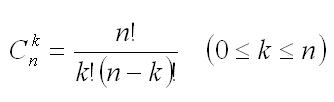Competitions

# Binomial coefficients 1

Time limit 1 second
Memory limit 128 MiB

Let n be a non-negative integer. Let n! = 1 * 2 *... * n (0! = 1) andYou are given the numbers n and k. Calculate C(n,k).

## Input data

The first line contains the number of test cases t (t50). Each of the next t lines contains two integers n and k (0n < 2^64, 0C(n,k) < 2^64).

## Output data

Print t lines, each contains the value C(n,k) for corresponding test.

## Examples

Input example #1
6
0 0
1 0
1 1
2 0
2 1
2 2

Output example #1
1
1
1
1
2
1

Author Anton Lunyov